Register for Maths, Science, English, Reasoning Olympiad Exams Register here | Book Free Demo for Live Olympiad Classes here | Check Olympiad Exam Dates here | Buy Practice Papers for IMO, IOM, HEO, IOEL etc here | Login here to participate in all India free mock test on every Saturday

# Geometry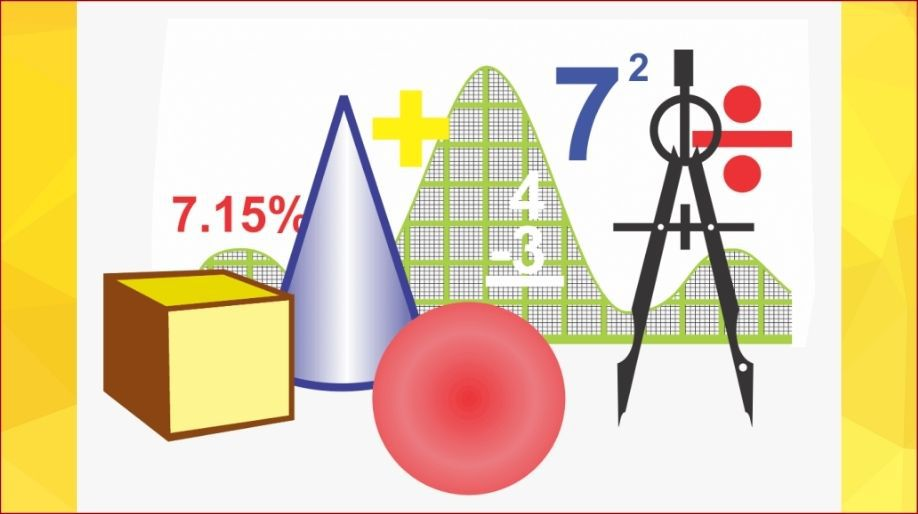• Geometry is the branch of mathematics that focuses on the measurement and relationship of lines, angles, surfaces, solids, and points.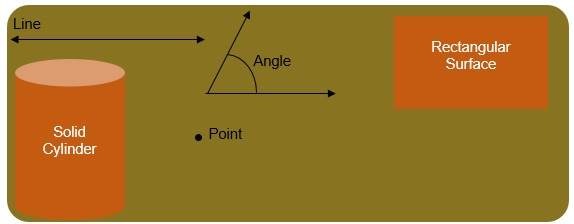# Angles

An angle is the combination of two rays with a common end-point.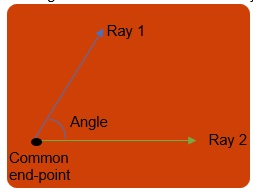• Angle is measured in degrees. A degree is denoted by °.

The types of angle are: acute angle, obtuse angle, right angle, complete angle, zero angle, reflex angle, and straight angle.
Acute Angle: An angle whose measure is less than 90 degrees but greater than 0 degrees.
Obtuse Angle: An angle whose measure is less than 180 degrees but greater than 90 degrees.
Right Angle: An angle whose measure is 90 degrees is called right angle.
Complete Angle: An angle whose measure is 360 degrees is called complete angle.
Zero Angle: If the measure of an angle is zero, its called zero angle.
Reflex Angle: An angle whose measure is greater than 180 degrees but less than 360 degrees is called reflex angle.
Straight Angle: An angle whose measure is 180 degrees is called straight angle.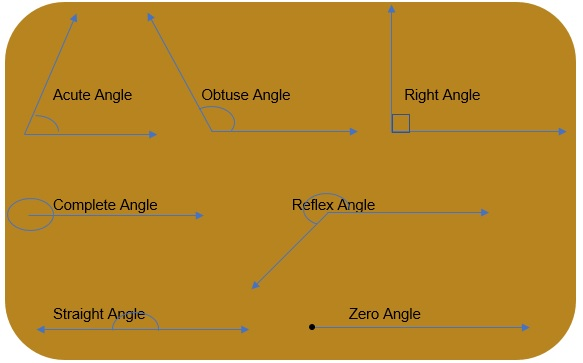Note: 360 degrees angle and 0 degree angle are different. In 360 degrees, there is one full rotation of one ray with respect to another. While in zero angle, there is no such rotation. Both rays in 0 degree angle lie on the same plane.

Construction of a 20 degree angle using ruler and compass.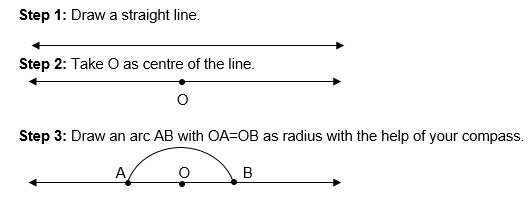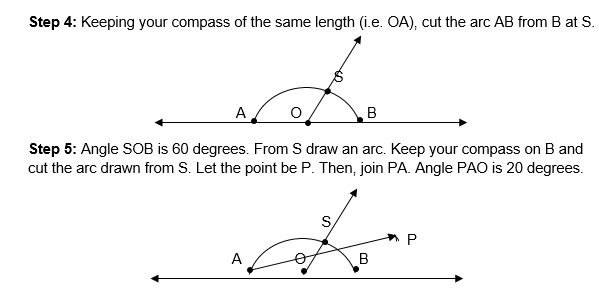## Lines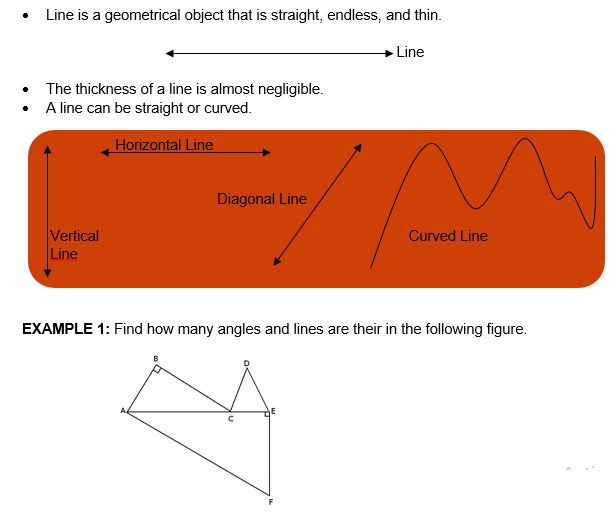SOLUTION:
Angles are= ABC, BCA, BAC, CDE, DEC, ECD, AEF, EFA, FAE
Lines are= AB, BC, CD, DE, EF, FA, AC, CE

# Circle

• A circle is a shape with all points the same distance from its centre. Area of a circle= X R2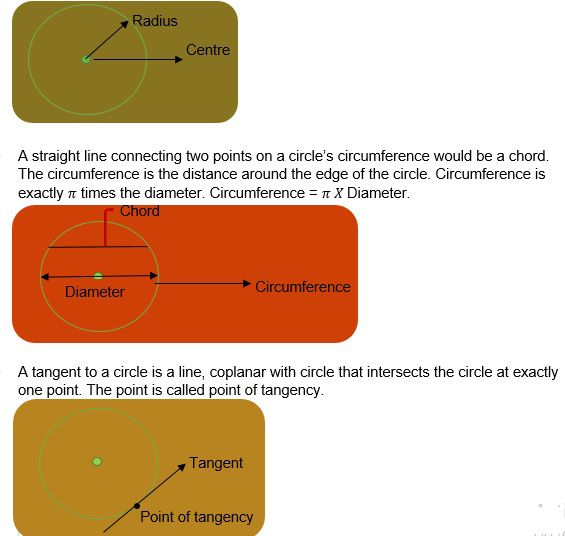## Triangles

• A triangle is a plane figure with three straight sides and three angles.
• On the basis of sides, triangles are classified into: Scalene (all sides are different), Isosceles (Two sides are equal), Equilateral (All sides are equal)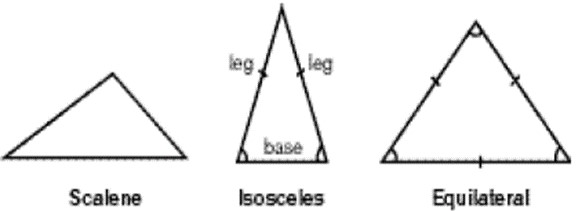• On the basis of angels, triangles are divided into: acute(all angles are acute), right(one angle is 90 degree), Obtuse(one angle is obtuse), Equiangular(all angles are equal).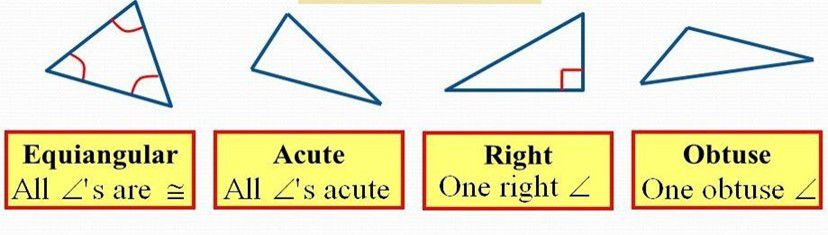## Cube

• In geometry, a cubeis a three-dimensional solid object bounded by six square faces, sides, with three meeting at each vertex.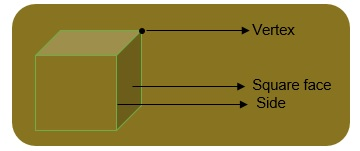EXAMPLE 1: The radius of the circle is 3 cm. Find its diameter, circumference, and area.

SOLUTION: Diameter= 2 X Radius = 2 X 3 = 6 cm

Circumference= 2 X X R = 2 X X 3= 18.84 cm Area= X R2 = X (3)2= 28.27 cm2

EXAMPLE 2: Find the number of triangles in the following figure and name them.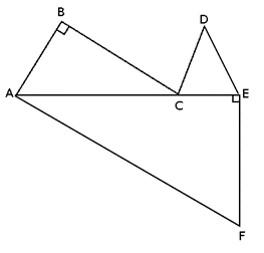SOLUTION:
Number of triangles= 3

They are= triangle ABC, triangle CDE, triangle AEF
triangle ABC= Right angle triangle
triangle CDE= Isosceles Triangle
triangle AEF= Right Angle Triangle

# Perimeter and Area of Squares and Rectangles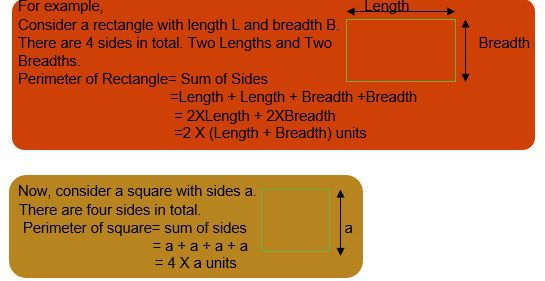• Area is a quantity that expresses the extent of a two-dimensional surface or shape in the plane.

Area of a rectangular surface= Length X Breadth units2
Area of a square surface= a X a = a2 units2

EXAMPLE 1: Find the area and perimeter of a rectangle with length 1cm and breadth 2cm

SOLUTION: Perimeter= 2 X (Length + Breadth) = 2 X (1+2)= 6 cm
Area= Length X Breadth= 1cm X 2cm= 2cm2

EXAMPLE 2: Find the area and perimeter of a square with side 3cm.

SOLUTION: Perimeter= 4 X 3 cm= 12cm
Area= 3cm X 3cm= 9cm2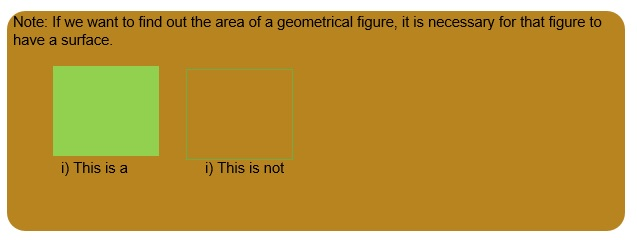# Practice these questions

Q1) Name the angles.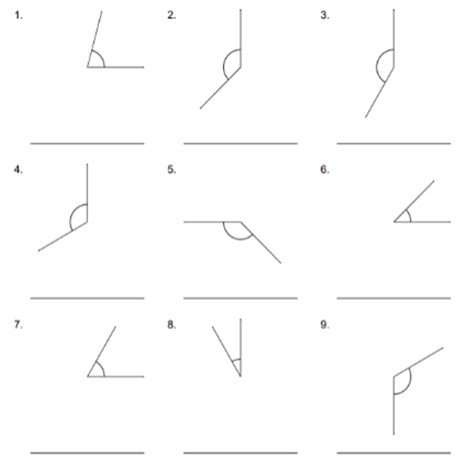Q2) Classify the triangles.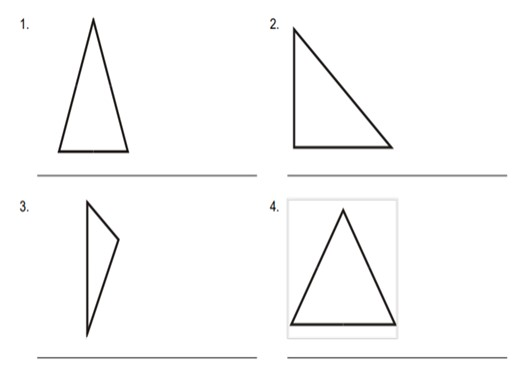Q3) Find the perimeter and area of the rectangle. (Hint: Divide the irregular rectangles into two regular rectangles.)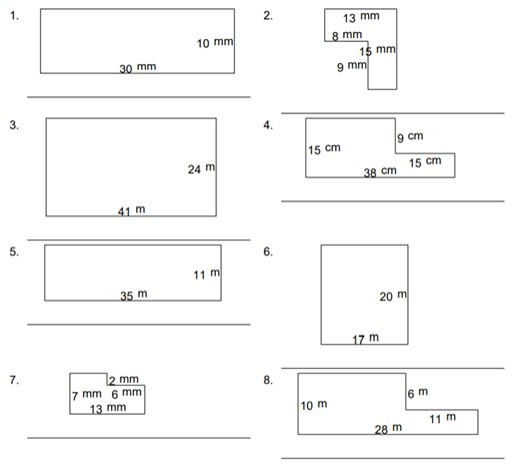Q4) Find the area and perimeter of the square below.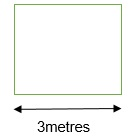# Recap

• Only a surface can have area.
• Perimeter of rectangle= 2 X (Length + Breadth)
Perimeter of square= 4 X a
• Area of rectangle= Length X Breadth
Area of square= A2
• Area of Circle=
• Circumference= 2 X X R
• Diameter = 2 X Radius
• There are 7 angles= acute, obtuse, reflex, complete, zero, right, and straight angle.
• On the basis of sides, there are 3 triangles= scalene, isosceles, equilateral triangle.
• On the basis of angle, there are 4 triangles = acute, obtuse, right, and equiangular triangle

## Quiz for Geometry

 Q.1 A room having a length of 15 ft. and breadth of 12 ft. has to be paved with marble leaving out a gap of 1 ft. along each edge. Find out the area that is to be paved with marble. a) 210 sq. ft. b) 125 sq. ft. c) 130 sq. ft. d) none
 Q.2 Find the perimeter and area.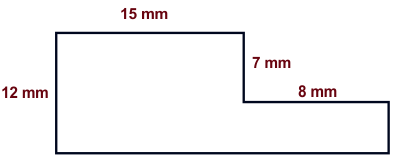a) 50mm, 100 mm2 b) 70mm, 220 mm2 c) 65mm,250 mm2 d) 75mm, 145 mm2
 Q.3 A square notepad has a area of 121 cm2. How long is each side of the notepad? a) 11cm b) 12cm c) 9.5cm d) 13cm
 Q.4 For a given perimeter, which statement is true? a) area of rectangle > area of square b) area of square > area of rectangle c) area of rectangle = area of square d) none
 Q.5 Find the perimeter and area.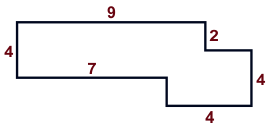a) 48m2, 34m b) 58m2, 42m c) 38m2, 28m d) 48m2, 37m
 Q.6 Find the perimeter and area.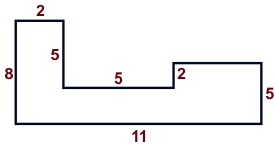a) 54 m2, 32 m b) 55 m2, 37 m c) 46 m2, 30 m d) 51 m2, 42 m
 Q.7 Mary wants new carpeting for her dining room. Her dining room is a 15 ft. by 12 ft. rectangle. How much carpeting does she need to buy to cover her entire dining room? a) 150 sq. ft. b) 280 sq. ft. c) 180 sq. ft. d) 110 sq. ft.
 Q.8 Vishal is making a display board for the school talent show. The display board is a 10 ft. by 9 ft. rectangle. If the ply board costs ₹ 12.5 per sq. foot, how much will it cost to make the display board? a) 1000 b) 1125 c) 1345 d) 900
 Q.9 Danny has a rectangular rose garden that measures 8 m by 10 m. One bag of fertilizer can cover 16 m2. How many bags will he need to cover the entire garden? a) 5 b) 6 c) 3 d) 4
 Q.10 Find the area of the blue coloured region.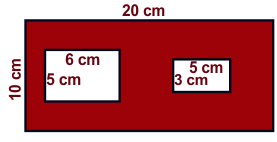a) 177sq. cm b) 145 sq. cm c) 155 sq. cm d) 166 sq. cm